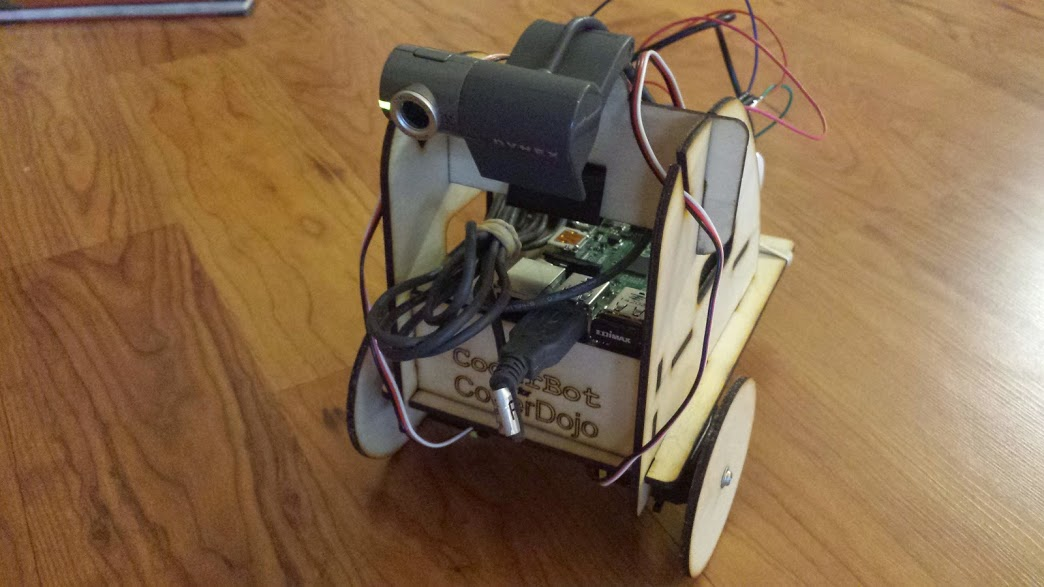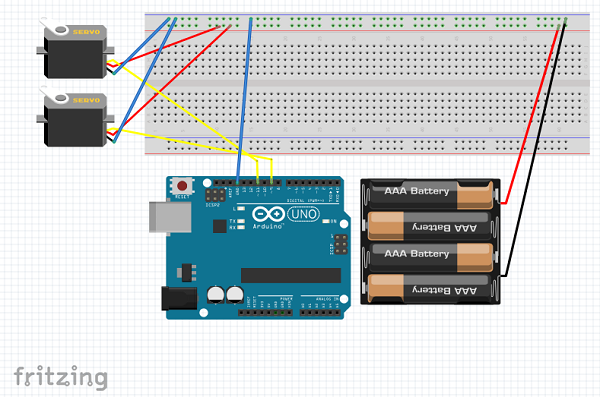# Drive Your Raspberry Pi Robot using Python Web APII’ve enjoyed building my own DIY Raspberry Pi robot and wanted to share the ideas behind building a remote control interface using the Flask python web framework.  This small HTTP based programming interface enabled me to build multiple apps to drive my robot.  You might enjoy using these ideas in your hobby robot design.

Here’s some background on the robot:

• The design uses a Raspberry PI 2
• The robot has a laser cut frame based on designs from CoderBot.org .
• The robot moves using two continuous rotation servos.  You can purchase similar servos through Amazon or Parallax.com.
• The Raspberry Pi is powered by a small cell phone battery charger.

The following diagram shows how the robot is wired using an Arduino.  Wiring to a Raspberry Pi is very similar.   Keep in mind that the signal wires for the left and right servo get connected to GPIO PINS 4 and 17 respectively on the Raspberry Pi.Building a HTTP programming interface using Flask

Let’s break down the major parts of the python script driving the robot.  This python script uses the following libraries:

• RPi.GPIO: This library enables the programmer to send digital signals to the input and output pins on the Raspberry PI.
• Flask: To enable the robot to accept HTTP requests from a web browser, we used a library known as Flask.

A continuous rotation servo has a simple protocol for controlling rotational

motion and speed using a frequency of voltage pulses.   This set of pulses is known as pulse width modulation. (PWM)  In the RPi.GPIO framework, the following code sets up pin 4 for PWM communication and stops the rotation of the servo.

``` #servo setup PIN_LEFT = 4 GPIO.setmode(GPIO.BCM) # Broadcom pin-numbering scheme GPIO.setup(PIN_LEFT, GPIO.OUT) # PWM pin set as output leftPWM = GPIO.PWM(PIN_LEFT,21.7) leftPWM.start(0) ```

``` ```

```#stop the servo leftPWM.ChangeDutyCycle(0) ```

To move the servo in one direction, you might do the following:

leftPWM.ChangeDutyCycle(5)

The code below initializes our pins 4 and 17 to send voltage pulses. The frequency of the voltage pulses on these pins will control the motion of the servos on the robot.

``` import RPi.GPIO as GPIO from flask import Flask, render_template import os```

``` PIN_LEFT = 4 PIN_RIGHT = 17 # Pin Setup: GPIO.setmode(GPIO.BCM) # Broadcom pin-numbering scheme GPIO.setup(PIN_LEFT, GPIO.OUT) # PWM pin set as output GPIO.setup(PIN_RIGHT, GPIO.OUT) ```

```leftPWM = GPIO.PWM(PIN_LEFT,21.7) rightPWM = GPIO.PWM(PIN_RIGHT,21.7 ) leftPWM.start(0) rightPWM.start(0) ```

Using the Flask python web framework, we create an instance of a flask application. We configure the root of the website(“/”) to return the template “driveRobot.html” This HTML file contains user interface elements and JavaScript that will make HTTP requests to the Flask server.

``` app = Flask(__name__)```

``` ```

```@app.route("/") def drive1(): return render_template("driveRobot.html") ```

The functions below show the process of sending out voltage frequencies to servos so that the robot can move forward, backward and stop. You can invoke these functions using HTTP requests.

``` @app.route("/forward") def forward(): leftPWM.ChangeDutyCycle(5) rightPWM.ChangeDutyCycle(1) return "forward"```

``` @app.route("/back") def back(): leftPWM.ChangeDutyCycle(1) rightPWM.ChangeDutyCycle(5) return "back" ```

```@app.route("/stop") def stop(): leftPWM.ChangeDutyCycle(0) rightPWM.ChangeDutyCycle(0) ```

The final lines run the web application.

``` if __name__ == "__main__": app.run(host='0.0.0.0') ```

To run this application, execute the following:

sudo python robotMove.py

Using a web browser, navigate to http://yourRaspberryPi:/

# Putting it all together

``` import RPi.GPIO as GPIO from flask import Flask, render_template import os```

``` PIN_LEFT = 4 PIN_RIGHT = 17 # Pin Setup: GPIO.setmode(GPIO.BCM) # Broadcom pin-numbering scheme GPIO.setup(PIN_LEFT, GPIO.OUT) # PWM pin set as output GPIO.setup(PIN_RIGHT, GPIO.OUT) leftPWM = GPIO.PWM(PIN_LEFT,21.7) rightPWM = GPIO.PWM(PIN_RIGHT,21.7 ) leftPWM.start(0) rightPWM.start(0) app = Flask(__name__) @app.route("/") def drive1(): return render_template("driveRobot.html") @app.route("/forward") def forward(): leftPWM.ChangeDutyCycle(5) rightPWM.ChangeDutyCycle(1) return "forward" @app.route("/back") def back(): leftPWM.ChangeDutyCycle(1) rightPWM.ChangeDutyCycle(5) return "back" @app.route("/stop") def stop(): leftPWM.ChangeDutyCycle(0) rightPWM.ChangeDutyCycle(0) return "stop" @app.route("/left") def left(): leftPWM.ChangeDutyCycle(1) rightPWM.ChangeDutyCycle(1) return "left" @app.route("/right") def right(): leftPWM.ChangeDutyCycle(5) rightPWM.ChangeDutyCycle(5) return "right" ```

```if __name__ == "__main__": app.run(host='0.0.0.0') ```

Top Stories

This entry was posted in open source, programming, stem, technology. Bookmark the permalink.